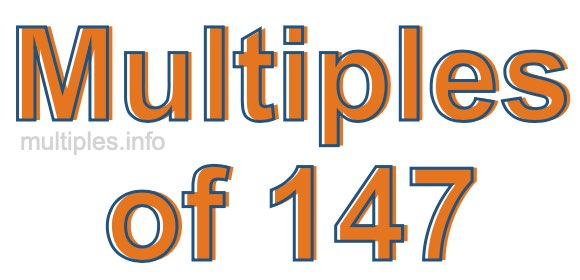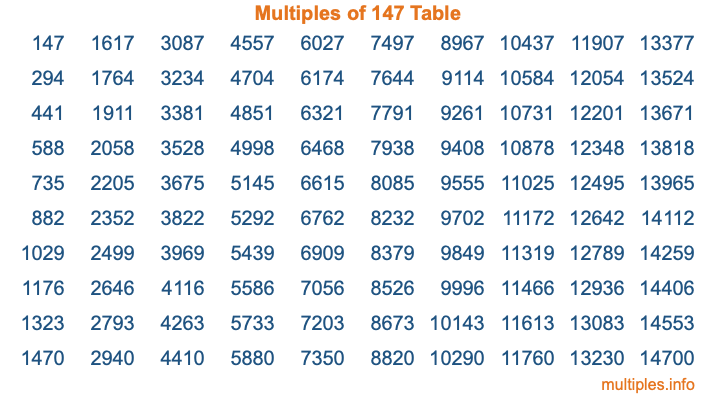Multiples of 147Welcome to the Multiples of 147 page. Here we will first teach you everything you will ever need to know about the multiples of 147, and then give you a study guide summary of everything we taught you to make sure you remember it all. Use this page to look up facts and learn information about the multiples of 147. This page will make you a multiples of one hundred forty-seven expert!

Definition of Multiples of 147
Multiples of 147 are all the numbers that when divided by 147 equal an integer. Each of the multiples of 147 are called a multiple. A multiple of 147 is created by multiplying 147 by an integer.

Therefore, to create a list of multiples of 147, you start with 1 multiplied by 147, then 2 multiplied by 147, then 3 multiplied by 147, and so on for as long as you want. Thus, the list of the first five multiples of 147 is 147, 294, 441, 588, and 735. To see a larger list of multiples of 147, see the printable image of Multiples of 147 further down on this page. We also have a category where you can choose any nth multiple of 147.

Multiples of 147 Checker
The Multiples of 147 Checker below checks to see if any number of your choice is a multiple of 147. In other words, it checks to see if there is any number (integer) that when multiplied by 147 will equal your number. To do that, we divide your number by 147. If the the quotient is an integer, then your number is a multiple of 147.

Is  a multiple of 147?

Least Common Multiple of 147 and ...
A Least Common Multiple (LCM) is the lowest multiple that two or more numbers have in common. This is also called the smallest common multiple or lowest common multiple and is useful to know when you are adding our subtracting fractions. Enter one or more numbers below (147 is already entered) to find the LCM.

Check out our LCM Calculator if you need more details about the Least Common Multiple or if you need the LCM for different numbers for adding and subtraction fractions.

nth Multiple of 147
As we stated above, 147 is the first multiple of 147, 294 is the second multiple of 147, 441 is the third multiple of 147, and so on. Enter a number below to find the nth multiple of 147.

th multiple of 147

Multiples of 147 vs Factors of 147
147 is a multiple of 147 and a factor of 147, but that is where the similarities end. All postive multiples of 147 are 147 or greater than 147. All positive factors of 147 are 147 or less than 147.

Below is the beginning list of multiples of 147 and the factors of 147 so you can compare:

Multiples of 147: 147, 294, 441, 588, 735, etc.

Factors of 147: 1, 3, 7, 21, 49, 147

As you can see, the multiples of 147 are all the numbers that you can divide by 147 to get a whole number. The factors of 147, on the other hand, are all the whole numbers that you can multiply by another whole number to get 147.

It's also interesting to note that if a number (x) is a factor of 147, then 147 will also be a multiple of that number (x).

Multiples of 147 vs Divisors of 147
The divisors of 147 are all the integers that 147 can be divided by evenly. Below is a list of the divisors of 147.

Divisors of 147: 1, 3, 7, 21, 49, 147

The interesting thing to note here is that if you take any multiple of 147 and divide it by a divisor of 147, you will see that the quotient is an integer.

Multiples of 147 Table
Below is an image of the first 100 multiples of 147 in a table. The table is in chronological order, column by column. The first column has the first ten multiples of 147, the second column has the next ten multiples of 147, and so on.The Multiples of 147 Table is also referred to as the 147 Times Table or Times Table of 147. You are welcome to print out our table for your studies.

Negative Multiples of 147
Although not often discussed or needed in math, it is worth mentioning that you can make a list of negative multiples of 147 by multiplying 147 by -1, then by -2, then by -3, and so on, to get the following list of negative multiples of 147:

-147, -294, -441, -588, -735, etc.

Multiples of 147 Summary
Below is a summary of important Multiples of 147 facts that we have discussed on this page. To retain the knowledge on this page, we recommend that you read through the summary and explain to yourself or a study partner why they hold true.

There are an infinite number of multiples of 147.

A multiple of 147 divided by 147 will equal a whole number.

147 divided by a factor of 147 equals a divisor of 147.

The nth multiple of 147 is n times 147.

The largest factor of 147 is equal to the first positive multiple of 147.

147 is a multiple of every factor of 147.

147 is a multiple of 147.

A multiple of 147 divided by a divisor of 147 equals an integer.

147 divided by a divisor of 147 equals a factor of 147.

Any integer times 147 will equal a multiple of 147.

Multiples of a Number
Here you can get the multiples of another number, all with the same attention to detail as we did for multiples of 147 on this page.

Multiples of
Multiples of 148
Did you find our page about multiples of one hundred forty-seven educational? Do you want more knowledge? Check out the multiples of the next number on our list!